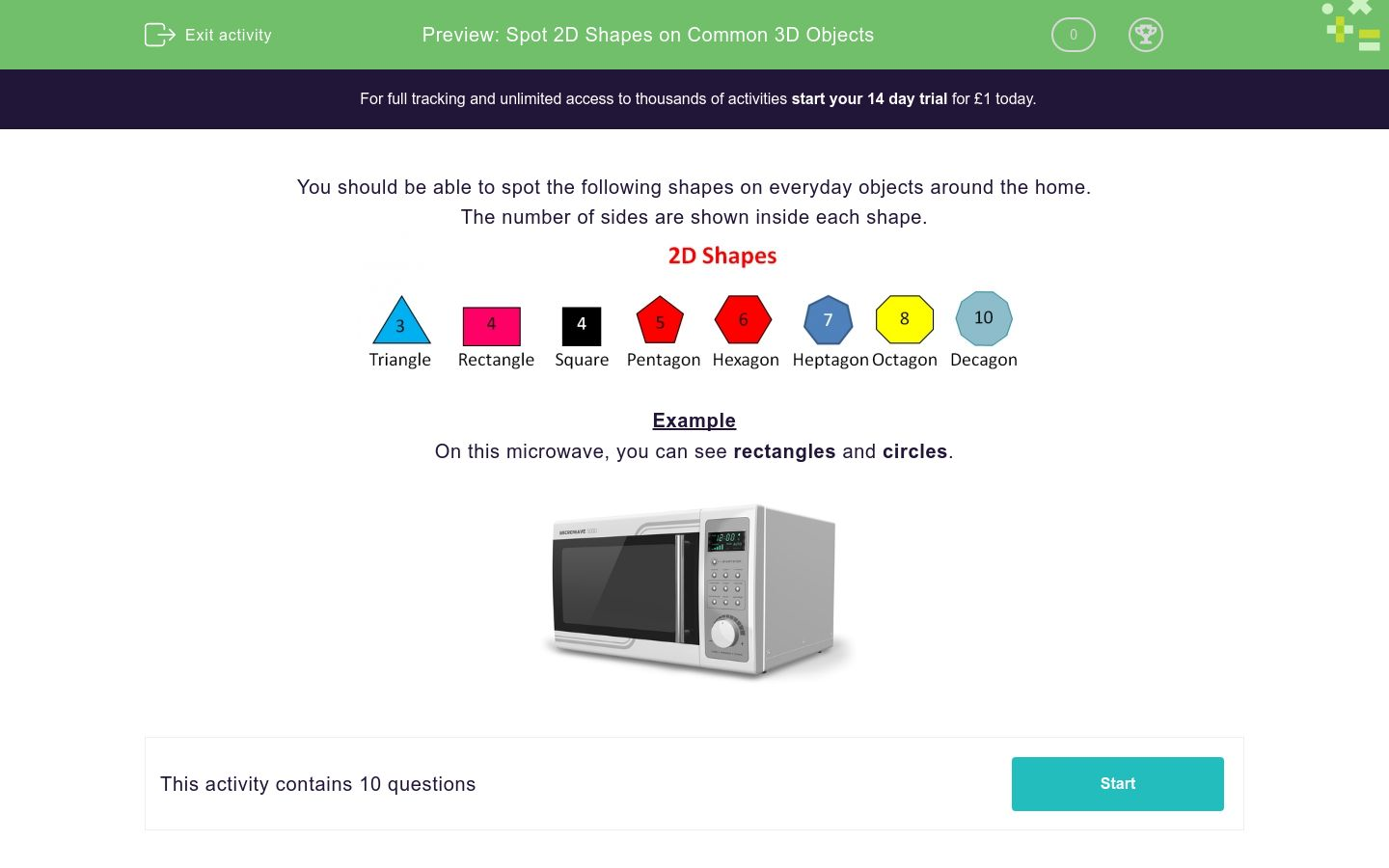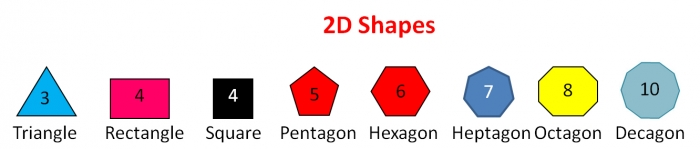# Spot 2D Shapes on Common 3D Objects

In this worksheet, students identify 2D shapes found on real 3D objects.Key stage:  KS 1

Curriculum topic:   Geometry: Properties of Shapes

Curriculum subtopic:   Identify 2D with 3D Shapes

Difficulty level:### QUESTION 1 of 10

You should be able to spot the following shapes on everyday objects around the home.

The number of sides are shown inside each shape.Example

On this microwave, you can see rectangles and circles.Here is a cardboard box.

What shapes can you see on its faces?triangles

rectangles

circles

pentagons

Here are some cupboard doors.

What shapes can you see on the drawing?squares

triangles

circles

rectangles

Here is a tin can.

What flat shapes can you see on its faces?circles

rectangles

squares

triangles

Here is a magnifying glass.

Which shape can be found around the lens?triangle

square

rectangle

circle

Here is a tennis ball.

What flat shapes can you see on its faces?circle

triangle

square

rectangle

none of these

Here is a washing machine.

What flat shapes can be found on the picture?triangles

pentagons

rectangles

circles

Here is a telephone box.

Which shapes can be found on its faces?triangles

squares

rectangles

circles

Here is a traffic sign.

Which shapes can be found on it?triangle

squares

rectangles

circles

Here are some floor tiles.

Which shapes can be found on the floor tiles?hexagons

squares

octagons

pentagons

Here is part of a honeycomb.

Which shapes can be found on the honeycomb?decagons

pentagons

octagons

hexagons

• Question 1

Here is a cardboard box.

What shapes can you see on its faces?rectangles
EDDIE SAYS
A cuboid is made of rectangles.
• Question 2

Here are some cupboard doors.

What shapes can you see on the drawing?circles
rectangles
EDDIE SAYS
The doors are rectangles.
The handles are circles.
• Question 3

Here is a tin can.

What flat shapes can you see on its faces?circles
EDDIE SAYS
A cylinder has a circle at the top and at its base.
• Question 4

Here is a magnifying glass.

Which shape can be found around the lens?circle
EDDIE SAYS
The lens is circular.
• Question 5

Here is a tennis ball.

What flat shapes can you see on its faces?none of these
EDDIE SAYS
There are no flat shapes on a sphere.
• Question 6

Here is a washing machine.

What flat shapes can be found on the picture?rectangles
circles
EDDIE SAYS
There are lots of rectangles on its faces.
The door is circular and so is the control button.
• Question 7

Here is a telephone box.

Which shapes can be found on its faces?rectangles
EDDIE SAYS
It is made of lots of rectangles, with a curved top.
• Question 8

Here is a traffic sign.

Which shapes can be found on it?triangle
rectangles
circles
EDDIE SAYS
The traffic light is made of circles and a rectangle.
The sign is triangular.
• Question 9

Here are some floor tiles.

Which shapes can be found on the floor tiles?squares
octagons
EDDIE SAYS
The grey tiles are squares.
The orange tiles are octagons.
• Question 10

Here is part of a honeycomb.

Which shapes can be found on the honeycomb?hexagons
EDDIE SAYS
A honeycomb is made of hexagons.
---- OR ----

Sign up for a £1 trial so you can track and measure your child's progress on this activity.

### What is EdPlace?

We're your National Curriculum aligned online education content provider helping each child succeed in English, maths and science from year 1 to GCSE. With an EdPlace account you’ll be able to track and measure progress, helping each child achieve their best. We build confidence and attainment by personalising each child’s learning at a level that suits them.

Get started